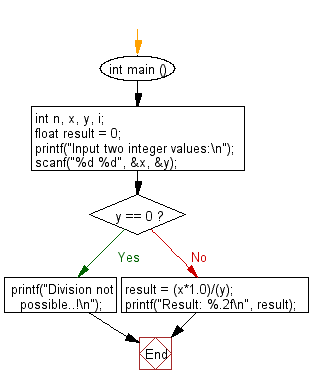﻿ C : Read 2 integers and dividing the first number by second

# C Exercises: Read two integers and dividing the first number by second

## C Basic Declarations and Expressions: Exercise-117 with Solution

Write a C program that reads two integers and divides the first number by second, print the result of this division with two digits after the decimal point and prints "Division not possible..!" if the division is not possible.

Sample Solution:

C Code:

``````#include <stdio.h>

int main () {
int n, x, y, i;
float result = 0;

// Prompt user for input
printf("Input two integer values:\n");

// Read two integer values from user and store them in 'x' and 'y'
scanf("%d %d", &x, &y);

// Check if 'y' is zero (division by zero case)
if (y == 0) {
printf("Division not possible..!\n"); // Print an error message
}
else {
// Calculate the result of division as a float value
result = (x * 1.0) / (y);

// Print the result with two decimal places
printf("Result: %.2f\n", result);
}

return 0; // End of program
}
``````

Sample Output:

```Input two integer values:
75
5
Result: 15.00
```

Flowchart:C programming Code Editor:

What is the difficulty level of this exercise?

Test your Programming skills with w3resource's quiz.

﻿